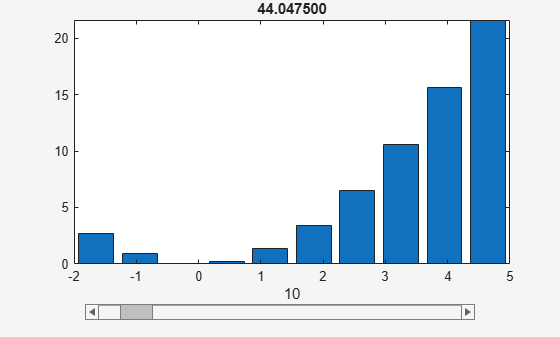# rsums

Interactive evaluation of Riemann sums

## Syntax

``rsums(f)``
``rsums(f,a,b)``
``rsums(f,[a,b])``

## Description

example

````rsums(f)` interactively approximates the integral of f(x) by middle Riemann sums for x from 0 to 1. `rsums(f)` displays a graph of f(x) using 10 terms (rectangles). You can adjust the number of terms taken in the middle Riemann sum by using the slider below the graph. The number of terms available ranges from 2 to 128. `f` can be a character vector or a symbolic expression. The height of each rectangle is determined by the value of the function in the middle of each interval.```

example

````rsums(f,a,b)` and `rsums(f,[a,b])` approximates the integral for x from `a` to `b`.```

## Examples

collapse all

Approximate the integral ${\int }_{0}^{1}{\mathit{x}}^{2}\text{\hspace{0.17em}}\mathrm{dx}$ by middle Riemann sum. `rsums` displays a graph of ${x}^{2}$ using 10 terms of the midpoint Riemann sum for the integration range from 0 to 1. The total sum is 0.3325.

```syms x rsums(x^2)```

Change the integration range of $x$ from -2 to 5. The total Riemann sum is 44.0475.

`rsums(x^2,-2,5)`## Input Arguments

collapse all

Integrand, specified as a symbolic expression, function, or number.

Lower bound, specified as a number or symbolic number.

Upper bound, specified as a number or symbolic number.

## Version History

Introduced before R2006a